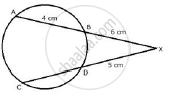Share

Chords Ab and Cd of a Circle When Extended Meet at Point X. Given Ab = 4 Cm, Bx = 6 Cm and Xd = 5 Cm, Calculate the Length of Cd. - ICSE Class 10 - Mathematics

ConceptTangent Properties - If a Chord and a Tangent Intersect Externally, Then the Product of the Lengths of Segments of the Chord is Equal to the Square of the Length of the Tangent from the Point of Contact to the Point of Intersection

Question

Chords AB and CD of a circle when extended meet at point X. Given AB = 4 cm, BX = 6 cm and XD = 5 cm, calculate the length of CD.

SolutionWe know that XB.XA = XD.XC
Or, XB.(XB + BA) = XD.(XD + CD)
Or, 6(6 + 4) = 5(5 + CD)
Or, 60 = 5(5 + CD)
Or, 5 + CD  = 60/5= 12
Or, CD = 12 − 5 = 7 cm.

Is there an error in this question or solution?

APPEARS IN

Solution Chords Ab and Cd of a Circle When Extended Meet at Point X. Given Ab = 4 Cm, Bx = 6 Cm and Xd = 5 Cm, Calculate the Length of Cd. Concept: Tangent Properties - If a Chord and a Tangent Intersect Externally, Then the Product of the Lengths of Segments of the Chord is Equal to the Square of the Length of the Tangent from the Point of Contact to the Point of Intersection.
S